• +91 9971497814
• info@interviewmaterial.com

# RD Chapter 14- Co-ordinate Geometry Ex-14.3 Interview Questions Answers

### Related Subjects

Question 1 : Find the coordinates of the point which divides the line segment joining (-1, 3) and (4, -7) internally in the ratio 3 : 4.

The line segment joining the points A (-1,3) and B (4, -7) is divided into the ratio 3 : 4
Let P (x, y) divides AB in the ratio 3 : 4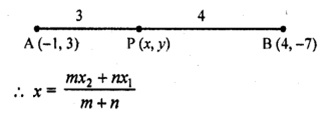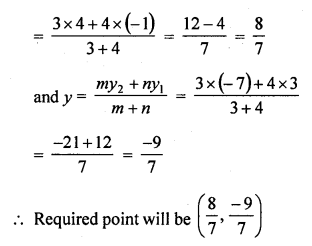Question 2 :
Find the points of trisection of the line segment joining the points :
(i) (5, -6) and (-7, 5)
(ii) (3, -2) and (-3, -4)
(iii) (2, -2) and (-7, 4) [NCERT]

(i) The line segment whose end points are A (5, -6) and B (-7,5) which is trisected at C and D
C divides it in the ratio 1 : 2
i.e., AC : CB = 1 : 2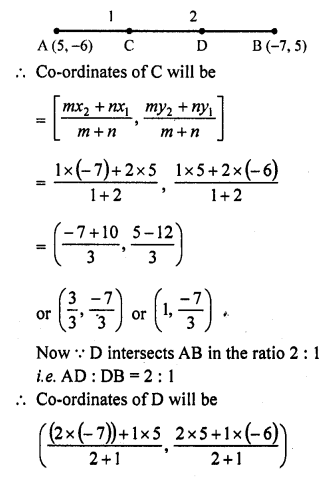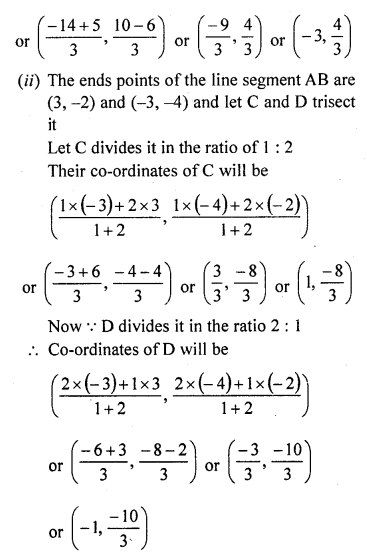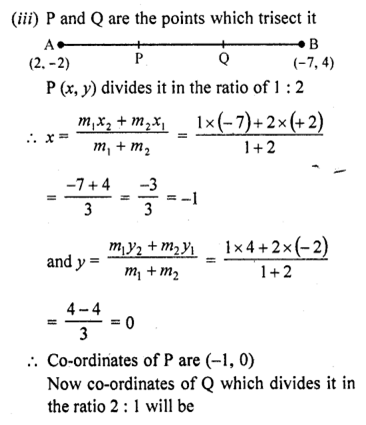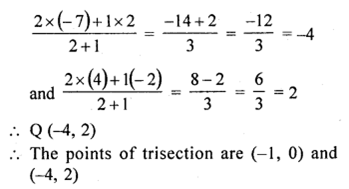Question 3 : Find the coordinates of the point where the diagonals of the parallelogram formed by joining the points (-2, -1), (1, 0) (4,3) and (1, 2) meet.

Answer 3 : Let the vertices of the parallelogram ABCD be A (-2, -1), B (1, 0), C (4, 3) and D (1, 2) in which AC and BD are its diagonals which bisect each other at O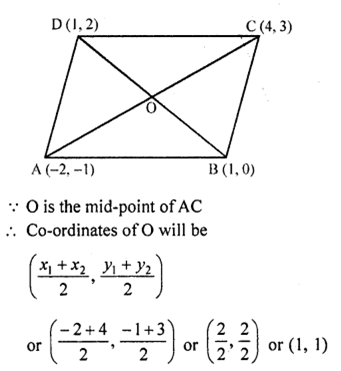Question 4 : Prove that the points (3, -2), (4, 0), (6, -3) and (5, -5) are the vertices of a parallelogram.

Let the vertices of the quadrilateral ABCD be A (3, -2), B (4, 0), C (6, -3) and D (5, -5)
Now co-ordinates of the mid-point of AC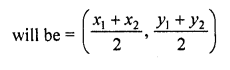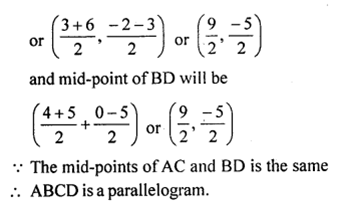Question 5 : If P (9a – 2, -b) divides the line segment joining A (3a + 1, -3) and B (8a, 5) in the ratio 3 : 1, find the values of a and b. [NCERT Exemplar]

Let P (9a – 2, -b) divides AB internally in the ratio 3 : 1.
By section formula,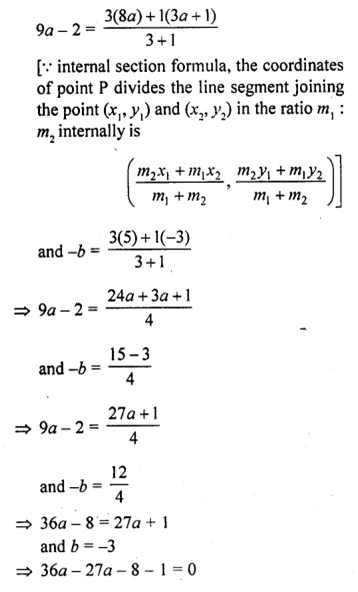=> 9a – 9 = 0
a = 1

Question 6 : If (a, b) is the mid-point of the line segment joining the points A (10, -6), B (k, 4) and a – 2b = 18, find the value of k and the distance AB. [NCERT Exemplar]

Answer 6 : Since, (a, b) is the mid-point of line segment AB.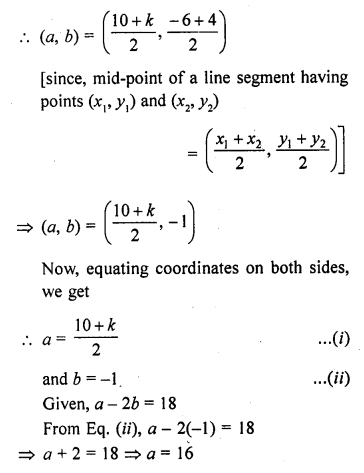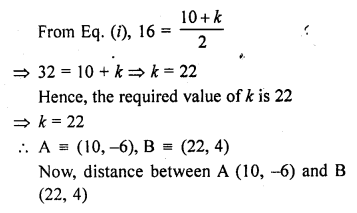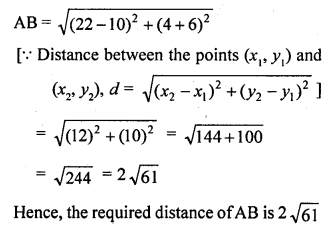Question 7 : Find the ratio in which the points (2, y) divides the line segment joining the points A (-2, 2) and B (3, 7). Also, find the value of y. (C.B.S.E. 2009)

Answer 7 : Let the point P (2, y) divides the line segment joining the points A (-2, 2) and B (3, 7) in the ratio m1 : m2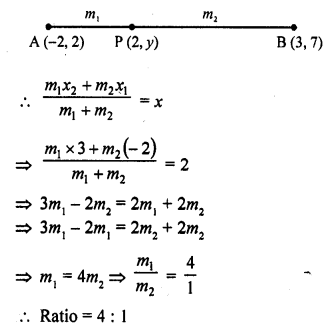Question 8 : If A (-1, 3), B (1, -1) and C (5, 1) are the vertices of a triangle ABC, find the length of the median through A.